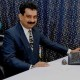# “The Knowledge Library”

Knowledge for All, without Barriers…

# “The Knowledge Library”

Knowledge for All, without Barriers……….

# Units of Measurements

Quantity – Units (S.I.)

●Length – Metre
●Time – Second
●Mass – Kilogram
●Area – Square meter
●Volume – Cubic meter
●Velocity – Metre/second
●Acceleration – Metre/second square
●Density – Kilogram/meter Cube
●Work – Joule
●Energy – Joule
●Force – Newton
●Pressure – Pascal or Newton/sq. meter Charge
●Frequency – Hertz
●Power – Watt
●Weight – Newton or Kilogram
●Impulse – Newton-second
●Angular velocity – Radian /second
●Viscosity – Poise
●Surface tension – Newton/square metre
●Heat – Joule
●Temperature – Kelvin
●Absolute temperature – Kelvin
●Resistance – Ohm
●Electric current – Ampere
●Electromotive force – Volt
●Electrical conductivity – Ohm/meter
●Electric energy – Kilowatt-hour
●Electric power – Kilowatt or watt
●Magnetic intensity – Orsted
●Coulomb – Electric Charge
●Magnetic induction – Gauss
●Luminous flux – Candela
●The intensity of sound – Decibel
●Power of lens – Dioptre
●Depth of sea – Fathom